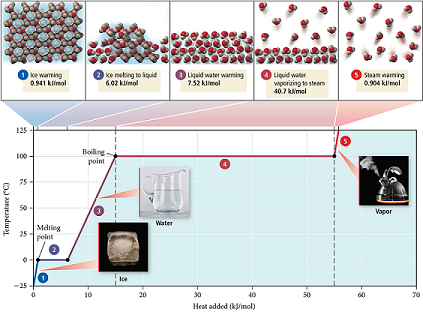# Problem: Refer to the figure below to answer each question.In moving from left to right along the line segment labeled 2 on the graph, heat is absorbed, but the temperature remains constant. Where does the heat go?

###### FREE Expert Solution

We have to determine why the temperature remains constant at segment marked 2 in the given diagram.

We can see that the segment marked 2 is at the melting point of water.

80% (222 ratings)###### Problem Details

Refer to the figure below to answer each question.In moving from left to right along the line segment labeled 2 on the graph, heat is absorbed, but the temperature remains constant. Where does the heat go?

Frequently Asked Questions

What scientific concept do you need to know in order to solve this problem?

Our tutors have indicated that to solve this problem you will need to apply the Heating and Cooling Curves concept. You can view video lessons to learn Heating and Cooling Curves. Or if you need more Heating and Cooling Curves practice, you can also practice Heating and Cooling Curves practice problems.

What professor is this problem relevant for?

Based on our data, we think this problem is relevant for Professor Coon's class at UNI.